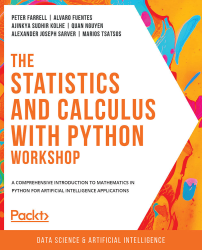•#### The Statistics and Calculus with Python Workshop#### Overview of this book

Are you looking to start developing artificial intelligence applications? Do you need a refresher on key mathematical concepts? Full of engaging practical exercises, The Statistics and Calculus with Python Workshop will show you how to apply your understanding of advanced mathematics in the context of Python. The book begins by giving you a high-level overview of the libraries you'll use while performing statistics with Python. As you progress, you'll perform various mathematical tasks using the Python programming language, such as solving algebraic functions with Python starting with basic functions, and then working through transformations and solving equations. Later chapters in the book will cover statistics and calculus concepts and how to use them to solve problems and gain useful insights. Finally, you'll study differential equations with an emphasis on numerical methods and learn about algorithms that directly calculate values of functions. By the end of this book, you’ll have learned how to apply essential statistics and calculus concepts to develop robust Python applications that solve business challenges.
Preface1. Fundamentals of PythonFree Chapter
2. Python's Main Tools for Statistics3. Python's Statistical Toolbox4. Functions and Algebra with Python5. More Mathematics with Python6. Matrices and Markov Chains with Python7. Doing Basic Statistics with Python8. Foundational Probability Concepts and Their Applications9. Intermediate Statistics with Python10. Foundational Calculus with Python11. More Calculus with Python12. Intermediate Calculus with PythonAppendix# Sequences and Series

If you were to participate in a TV show where the \$10,000 question was "Given the numbers 2, 4, 8, 16, and 32, what comes next in the sequence?", what would your best guess be? If your response is 64, then congratulations—you just came closer to understanding one of the key concepts in mathematical abstraction: that of a sequence. A sequence is, pretty much like in the ordinary sense of the word, a particular order in which things follow each other. Here, things are (in most cases) integers or real numbers that are related. The order of the elements matters. The elements are also called the members or terms of the sequence.

For example, in the preceding sequence of the TV show you participated in, every term stems from the number prior being multiplied by 2; there is no end in this sequence as there is no end in the number of terms (integer numbers) you can come up with. In other instances, elements in a sequence can appear more than once. Think...